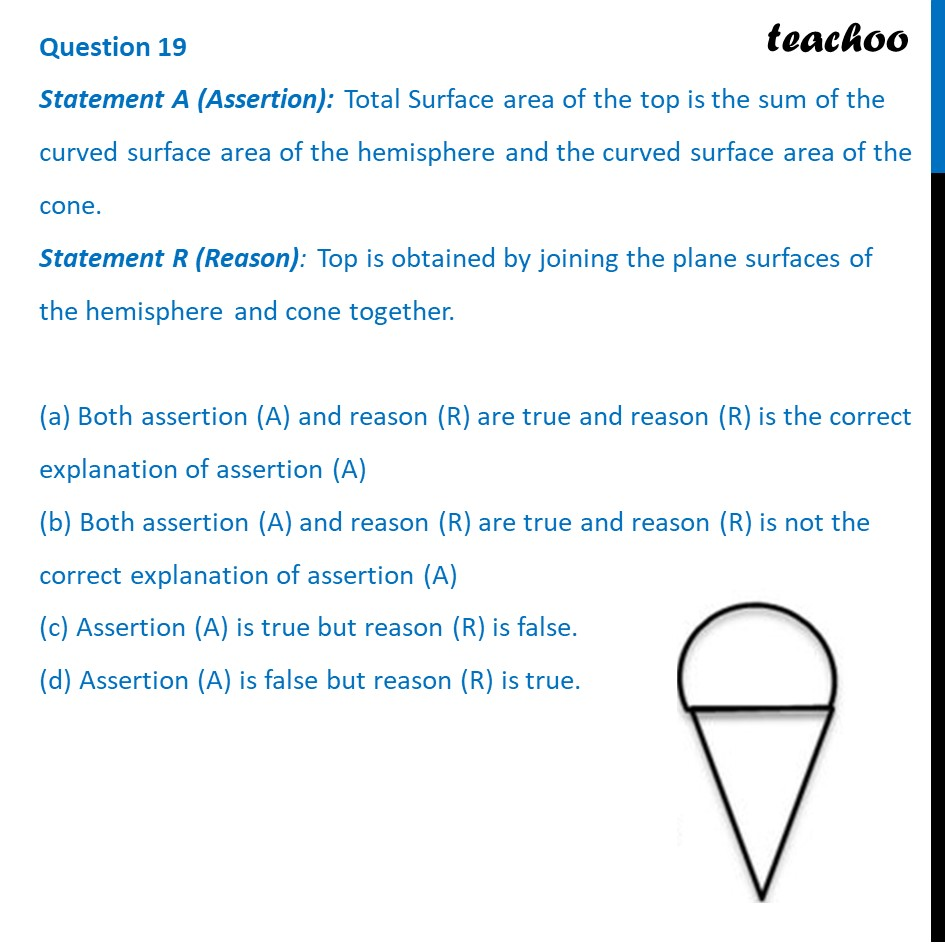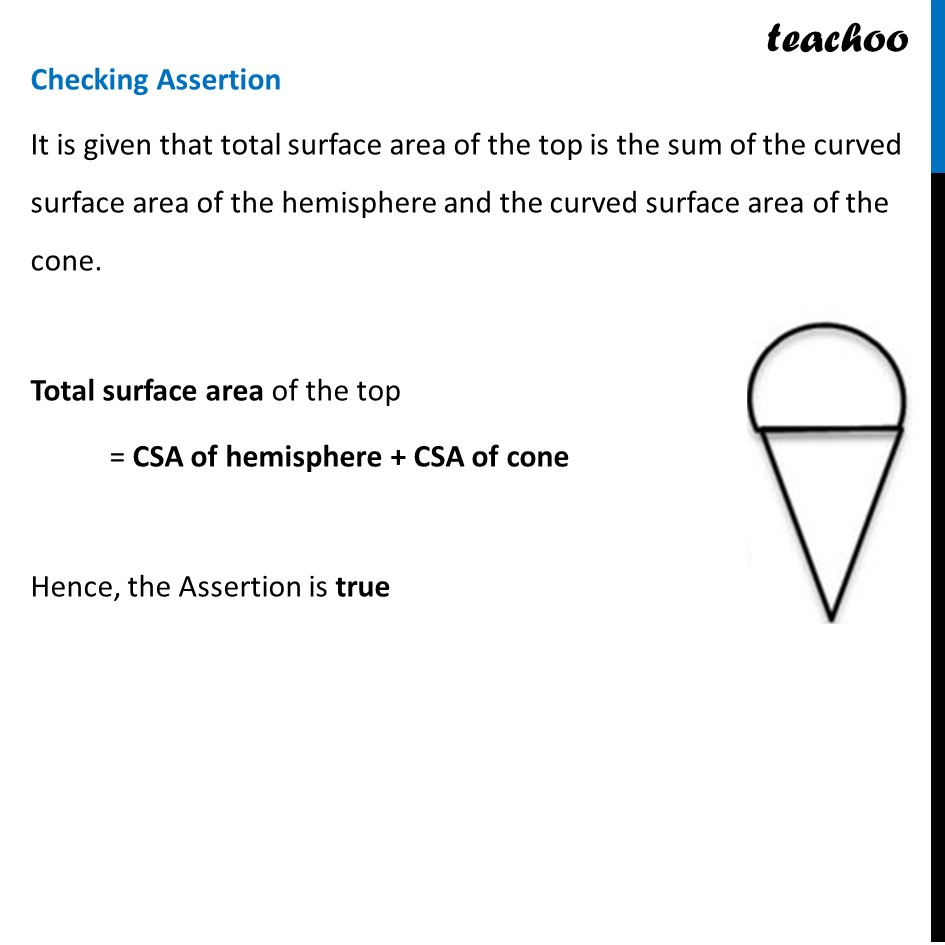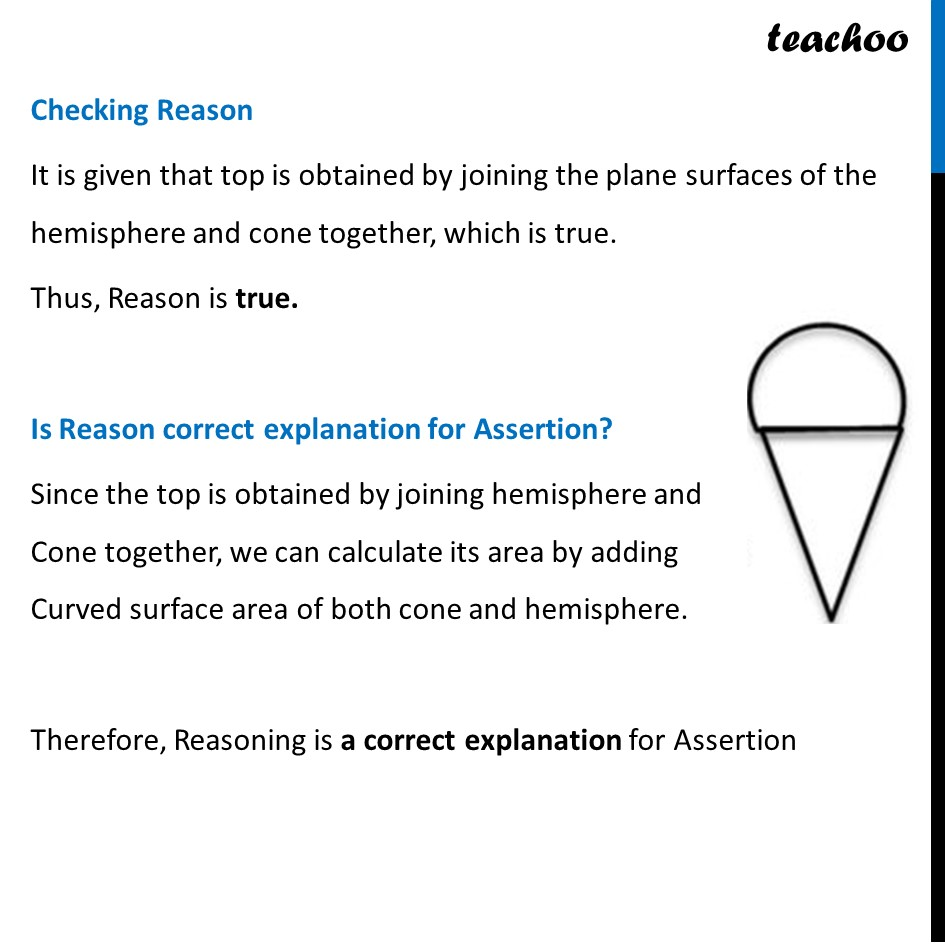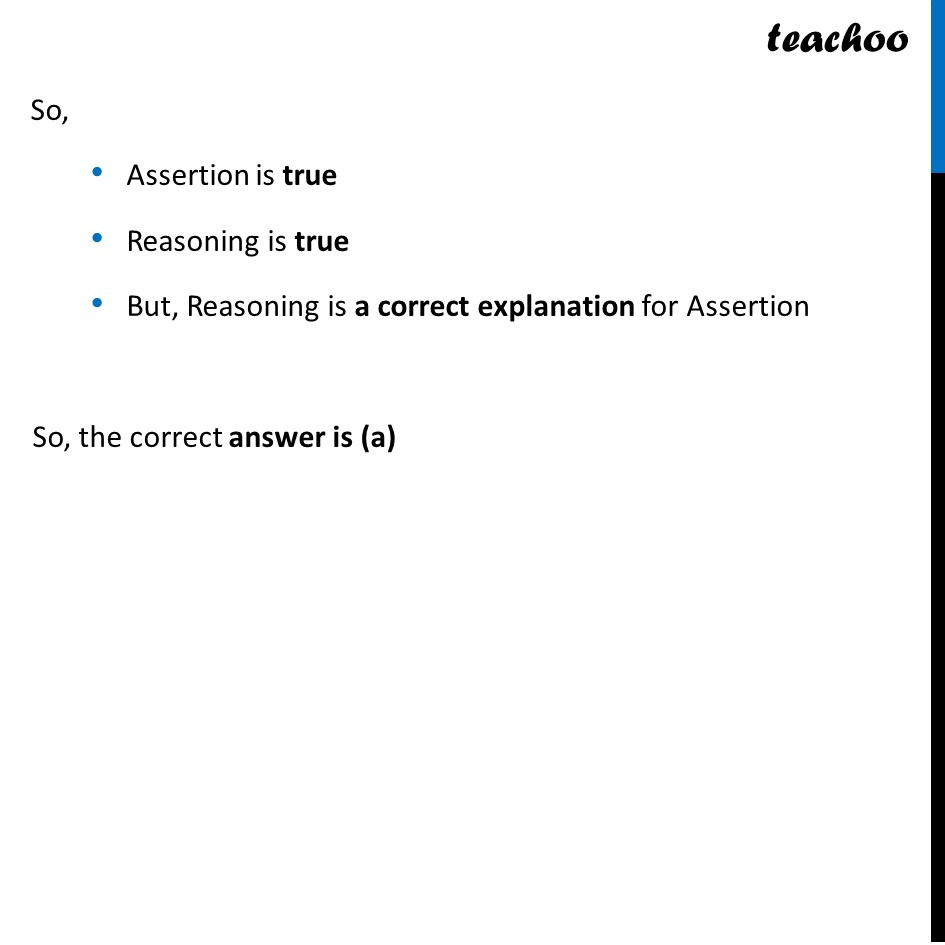CBSE Class 10 Sample Paper for 2024 Boards - Maths Standard

Class 10
Solutions of Sample Papers for Class 10 Boards

## (d) Assertion (A) is false but reason (R) is trueLearn in your speed, with individual attention - Teachoo Maths 1-on-1 Class

### Transcript

Checking Assertion It is given that total surface area of the top is the sum of the curved surface area of the hemisphere and the curved surface area of the cone. Total surface area of the top = CSA of hemisphere + CSA of cone Hence, the Assertion is true Checking Reason It is given that top is obtained by joining the plane surfaces of the hemisphere and cone together, which is true. Thus, Reason is true. Is Reason correct explanation for Assertion? Since the top is obtained by joining hemisphere and Cone together, we can calculate its area by adding Curved surface area of both cone and hemisphere. Therefore, Reasoning is a correct explanation for Assertion So, Assertion is true Reasoning is true But, Reasoning is a correct explanation for Assertion So, the correct answer is (a)